# Parallelograms

 1 Introduction to Parallelograms 2 What is a Parallelogram? 3 Types of Parallelograms 4 Properties of a Parallelogram 5 Parallelogram Theorems 6 Area of a Parallelogram 7 Solved Examples on Parallelograms 8 Thinking out of the Box! 9 Practice Questions on Parallelograms 10 Challenging Questions on Parallelograms 11 Maths Olympiad Sample Papers 12 Frequently Asked Questions (FAQs) 13 FREE Worksheets on Parallelograms

We at Cuemath believe that Math is a life skill. Our Math Experts focus on the “Why” behind the “What.” Students can explore from a huge range of interactive worksheets, visuals, simulations, practice tests, and more to understand a concept in depth.

Book a FREE trial class today! and experience Cuemath's LIVE Online Class with your child.

## Introduction to Parallelogram

A parallelogram is a special kind of quadrilateral

Rectangle, square, and rhombus are parallelogram examples.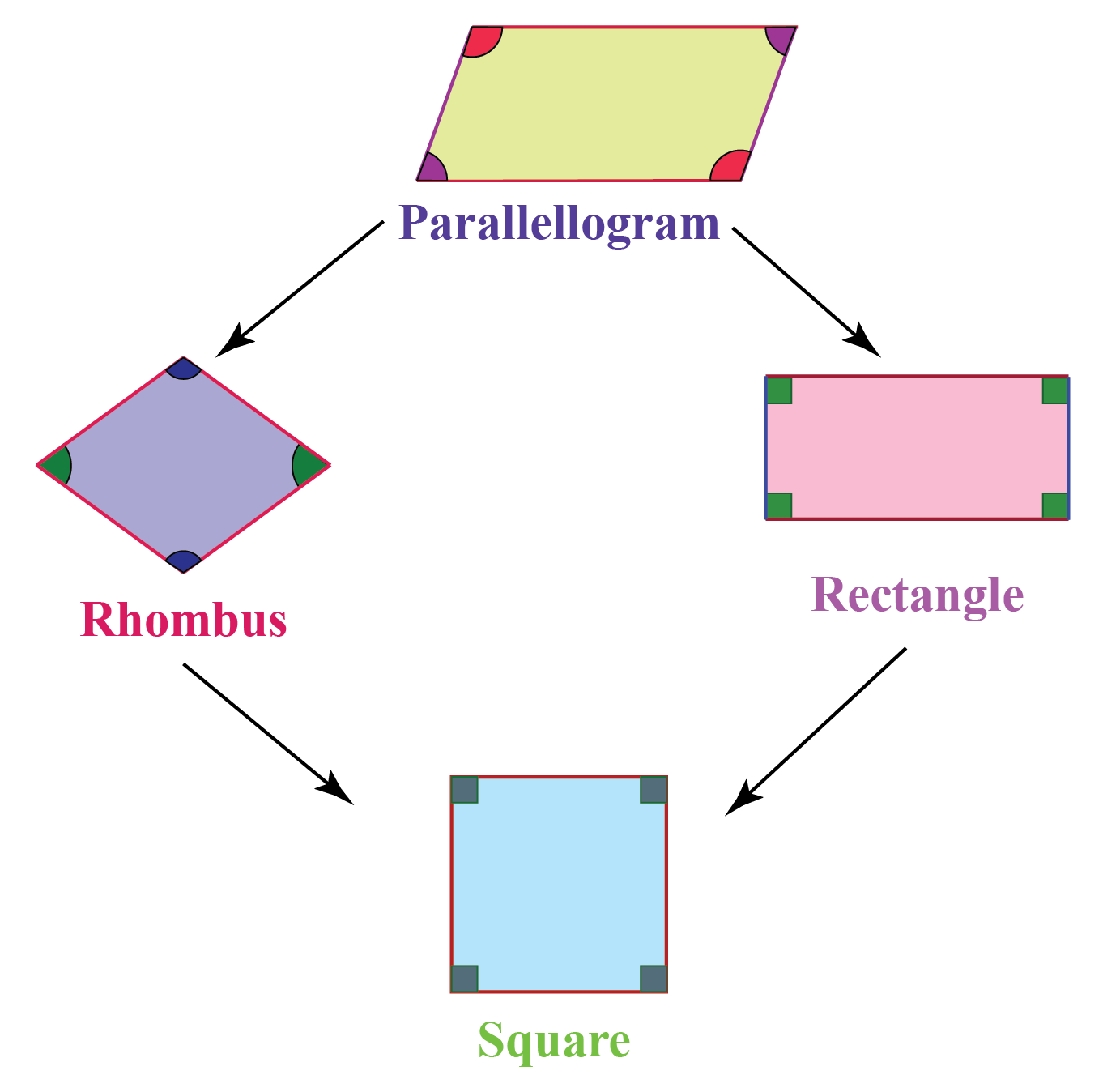### Parallelogram: Definition

A parallelogram is defined as a quadrilateral in which both pairs of opposite sides are parallel and equal.

## What is a Parallelogram?

A parallelogram is a convex polygon with $$4$$ edges and $$4$$ vertices.

It is a type of quadrilateral where the opposite sides are parallel and equal.

Drag the vertices to understand the relations between different elements of a parallelogram.

## Types of Parallelograms

Rhombuses, rectangles, and squares are common parallelogram examples.

The parallelogram properties for each are listed below.

### Rectangle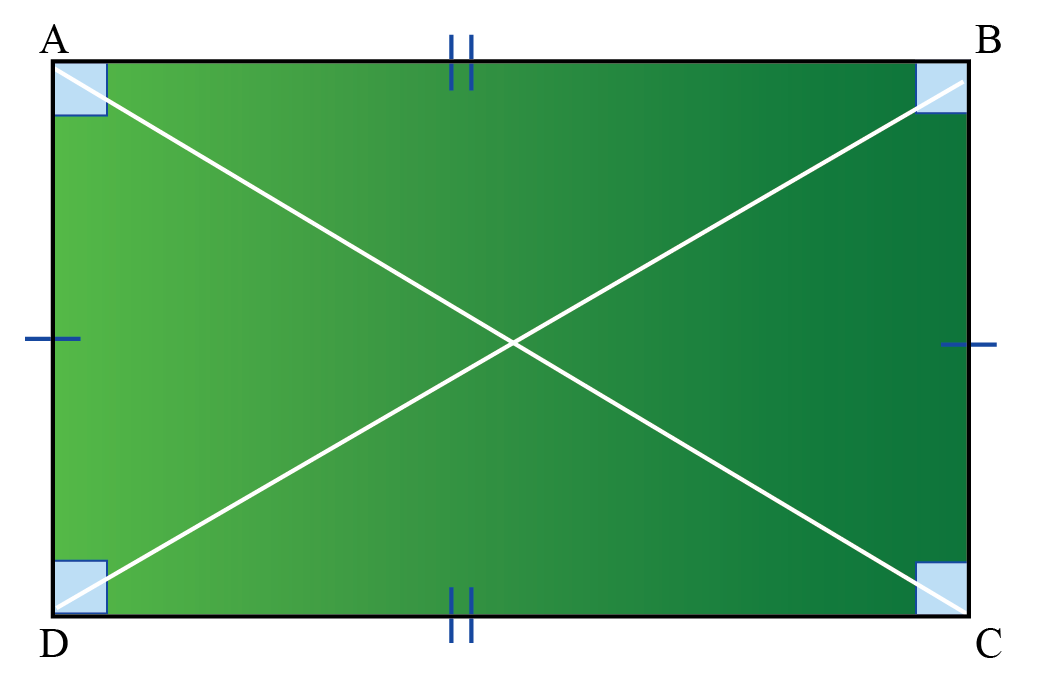A rectangle has:

• two pairs of parallel sides$A B\|D C \text{ and } A D\| B C$
• four right angles$\angle A=\angle B=\angle C=\angle D=90^{\circ}$
• opposite sides of equal lengths$A B=D C \text{ and } A D= B C$
• two equal diagonals$AC=BD$
• diagonals that bisect each other i.e., one diagonal divides the other diagonal into exactly two halves.

### Square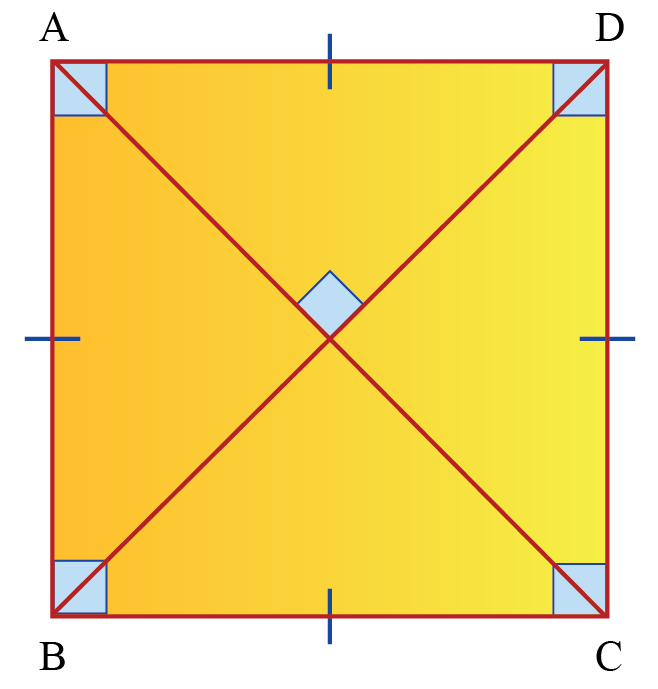A square has:

• four equal sides$AB=BC=CD=DA$
• four right angles$\angle A=\angle B=\angle C=\angle D=90^{\circ}$
• two pairs of parallel sides$A B\|D C \text { and } A D\| B C$
• two equal diagonals$AC=BD$
• diagonals that are perpendicular to each other$AC \perp BD$
• diagonals that bisect each other. i.e., one diagonal divides the other diagonal into exactly two halves.

### Rhombus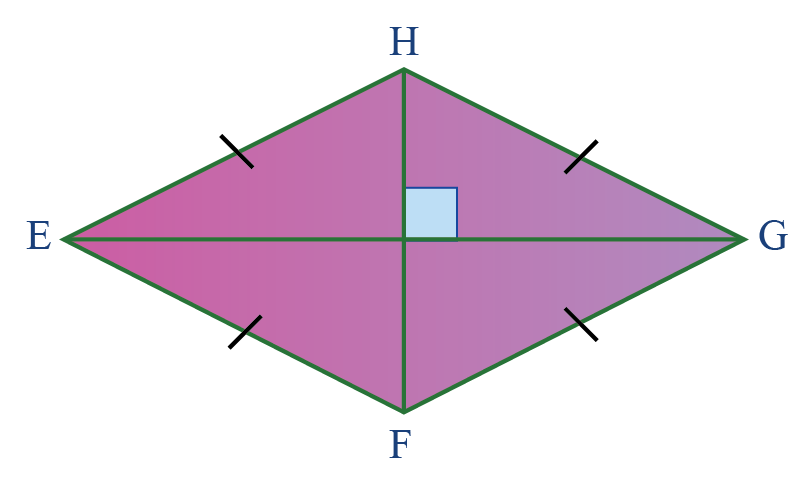A rhombus has:

• two pairs of parallel sides$E H\|F G \text{ and } E F\| H G$
• four equal sides$EH=HG=GF=FE$
• opposite angles are equal$\angle E = \angle G \text{ and } \angle H = \angle F$
• diagonals that are perpendicular to each other$EG \perp HF$
• diagonals that bisect each other. i.e., one diagonal divides the other diagonal into exactly two halves.

## Properties of a Parallelogram

Consider the parallelogram $$\text{PQRT}$$.

Let us understand the various parallelogram properties using $$\text{PQRT}$$.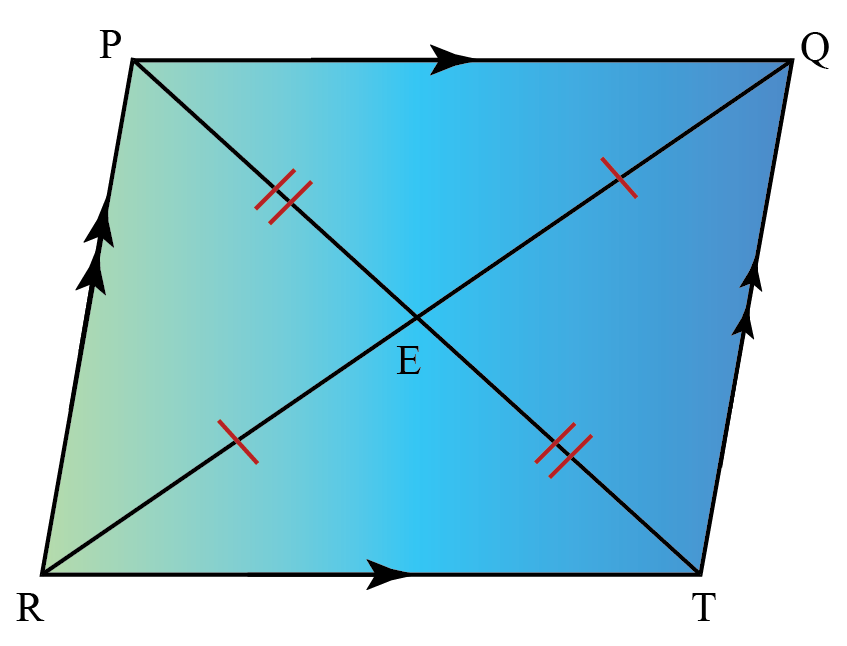• The opposites sides of a parallelogram are parallel.
$P Q\|R T \text{ and } PR\| Q T$
• Opposite sides of a parallelogram are equal.
$P Q=R T \text{ and } PR= Q T$
• Opposite angles of a parallelogram are equal.
$\angle P = \angle T \text{ and }\angle Q = \angle R$
• Diagonals of a parallelogram bisect each other. i.e., one diagonal divides the other diagonal into exactly two halves.
$R E=E Q \text{ and } PE= ET$
• Same-side interior angles supplements each other.
$\angle PRT + \angle RTQ =180 ^\circ$
$\angle RTQ + \angle TQP =180 ^\circ$
$\angle TQP + \angle QPR =180 ^\circ$
$\angle QPR + \angle PRT =180 ^\circ$
• Diagonals divide the parallelogram into two congruent triangles.
$\Delta \text{RPQ} \: \text{congruent to} \:\Delta \text{QTR}$
$\Delta \text{RPT} \: \text{congruent to}\: \Delta \text{QTP}$

## Parallelogram Theorems

### Theorem 1

In a parallelogram, opposite sides are equal. Conversely, if the opposite sides in a quadrilateral are equal, then it is a parallelogram.

Consider the following figure: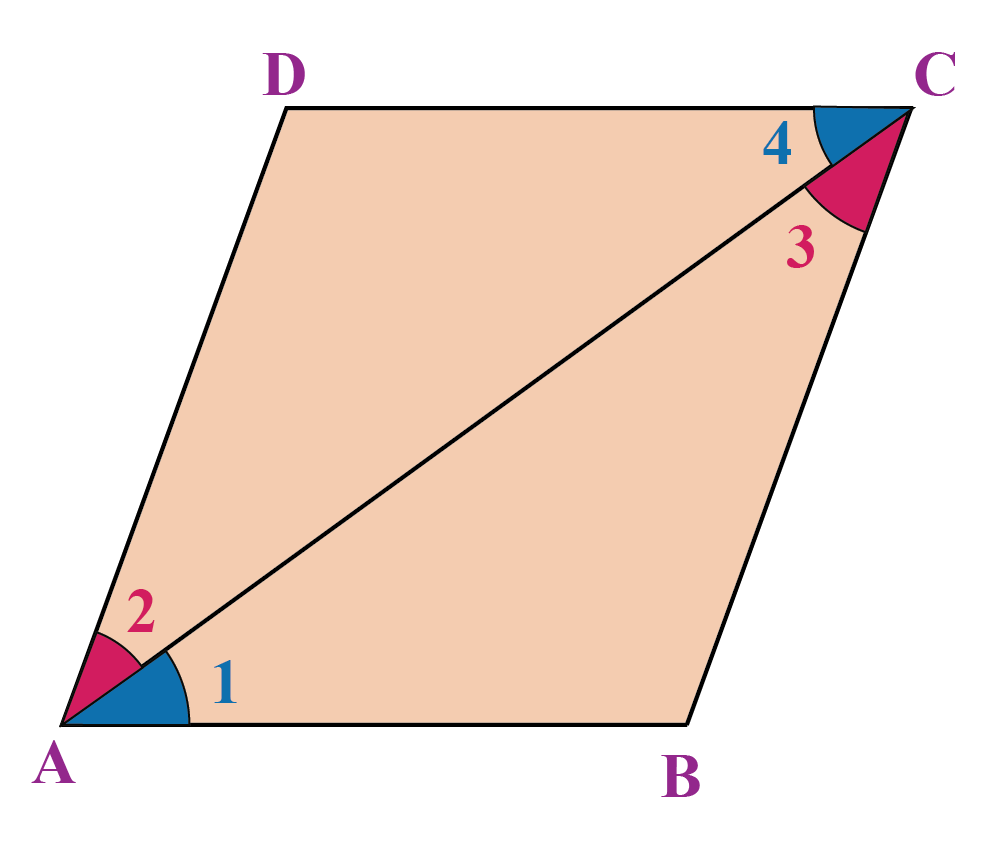Proof:

In $$\Delta ABC$$ and $$\Delta CDA$$,

\begin{align} AC&=AC \;(\text{common sides}) \\ \angle 1&=\angle 4 \;(\text{alternate interior angles}) \\ \angle 2&=\angle 3 \;(\text{alternate interior angles}) \end{align}

Thus, by the ASA criterion, the two triangles are congruent, which means that the corresponding sides must be equal.

Thus,

\begin{align}\boxed{AB=DC\;\text{and}\;AD=BC} \end{align}

This proves that opposite sides are equal in a parallelogram.

### Theorem 2

In a parallelogram, opposite angles are equal. Conversely, if the opposite angles in a quadrilateral are equal, then it is a parallelogram.

Consider the following figure: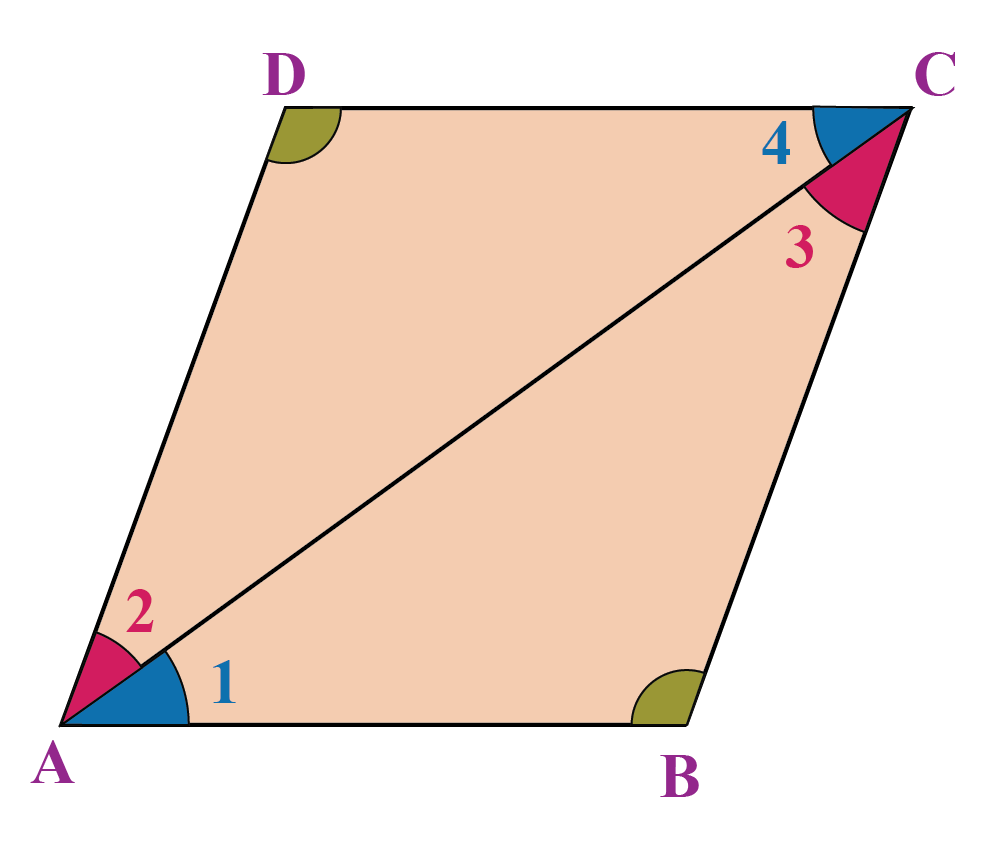Proof:

In $$\Delta ABC$$ and $$\Delta CDA$$,

\begin{align} AC&=AC\: ( \text{common sides} )\\ \angle 1&=\angle 4 \:(\text{alternate interior angles}) \\ \angle 2&=\angle 3 \: (\text{alternate interior angles} ) \end{align}

Thus, the two triangles are congruent, which means that

\begin{align}\boxed{\angle B=\angle D} \end{align}

Similarly, we can show that

\begin{align}\boxed{\angle A=\angle C} \end{align}

This proves that opposite angles in any parallelogram are equal.

### Theorem 3

In a parallelogram, the diagonals bisect each other. Conversely, if the diagonals in a quadrilateral bisect each other, then it is a parallelogram.

Consider the following figure: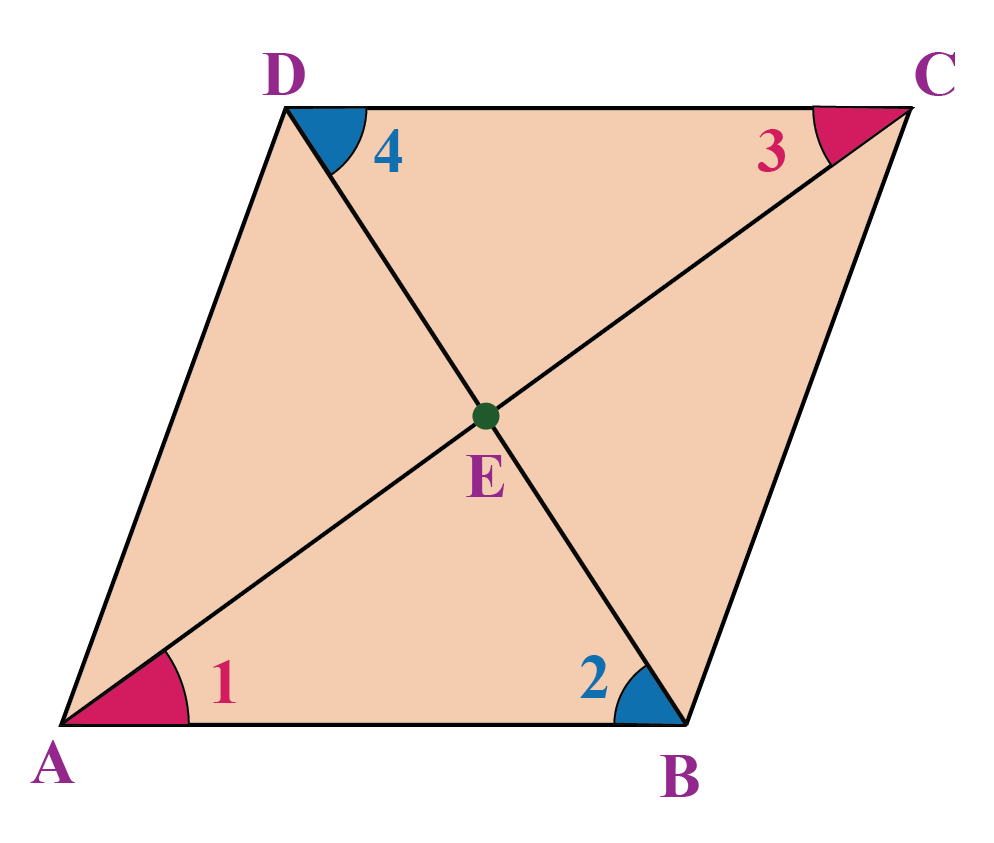Proof:

In $$\Delta AEB$$ and $$\Delta DEC$$, we have:

\begin{align} AB&=CD \:( \text{opposite sides of a parallelogram})\\ \angle 1&=\angle 3\:( \text{alternate interior angles}) \\ \angle 2&=\angle 4\:( \text{alternate interior angles}) \end{align}

By the ASA criterion, the two triangles are congruent, which means that

\begin{align}\boxed{AE=EC\;\text{and}\;BE=ED}\end{align}

Thus, the two diagonals bisect each other.

### Theorem 4

In a quadrilateral, if one pair of opposite sides are equal and parallel, then it is a parallelogram.

Consider the following figure: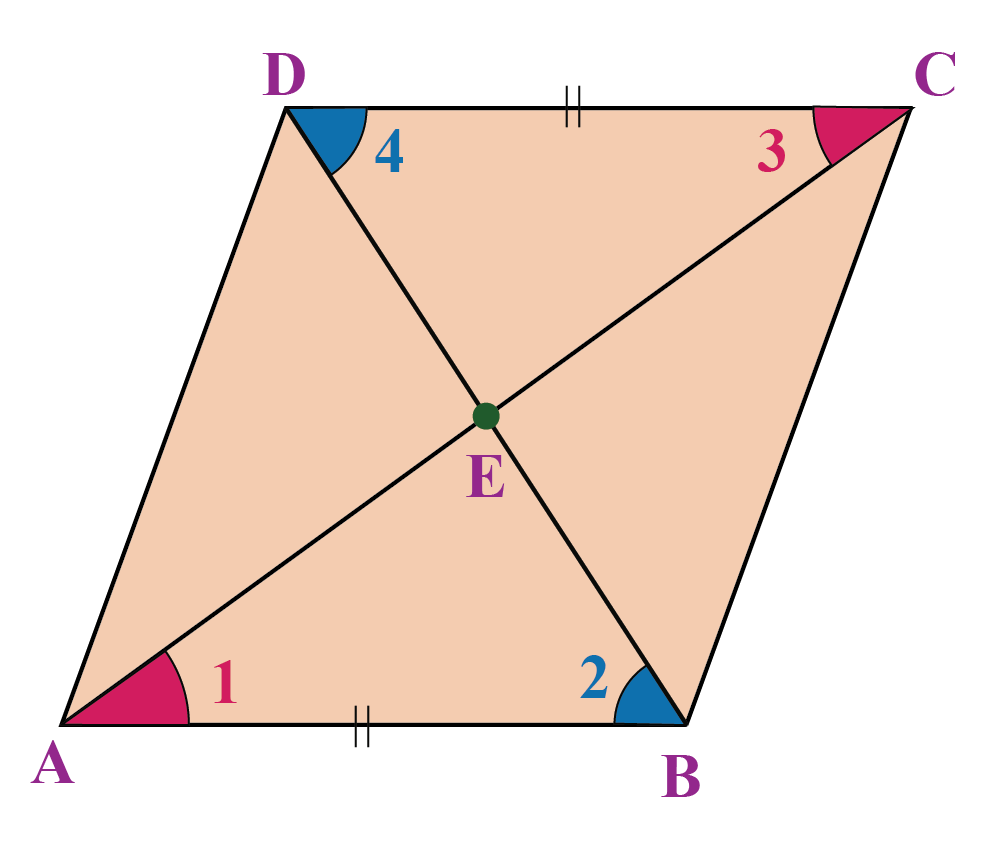It is given that $$AB=CD$$ and $$AB || CD$$.

We have to prove that ABCD is a parallelogram.

Proof:

In $$\Delta AEB$$ and $$\Delta DEC$$, we have:

\begin{align} \text{AB}&=\text{CD} \: \text{given} \\ \angle 1&=\angle 3\: \text{alternate interior angles} \\ \angle 2&=\angle 4\: \text{alternate interior angles} \end{align}

Thus, the two triangles are congruent, which means that:

\begin{align}\boxed{AE=EC\;\text{and}\;BE=ED}\end{align}

Therefore, the diagonals AC and BD bisect each other, and this further means that ABCD is a parallelogram.

## Area of a Parallelogram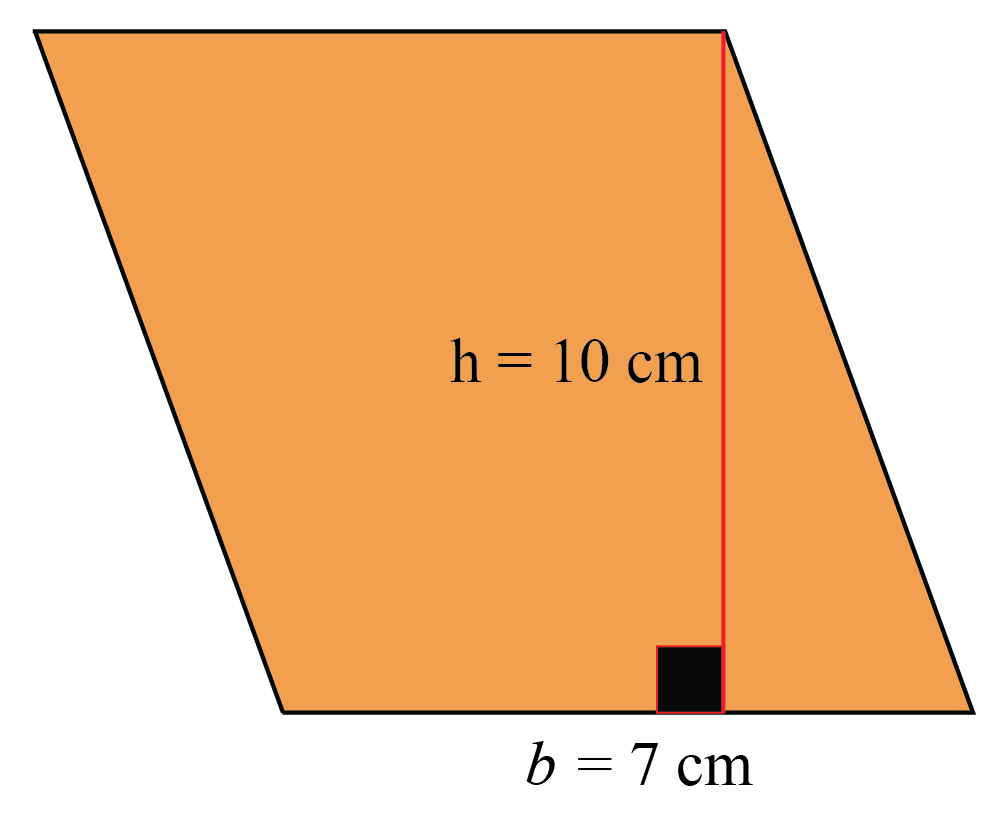Consider the parallelogram having base (b) and height (h).

Parallelogram area is given by the formula:

 $$\text{Area of Parallelogram} \!=\!\text{Base} \!\times\! \text{Height}$$

Input the base and height in the parallelogram area calculator shown below to calculate the area of a parallelogram.

Note: Parallelogram perimeter formula:

 $$2 \times$$ (Sum of length of adjacent sides)

Help your child score higher with Cuemath’s proprietary FREE Diagnostic Test. Get access to detailed reports, customized learning plans, and a FREE counseling session. Attempt the test now.

## Solved Examples

 Example 1

In the given parallelogram$$\text{ABCD}$$, $$\angle \text{A} = 90^\circ$$.

Show that all its angles are equal to $$90^\circ$$.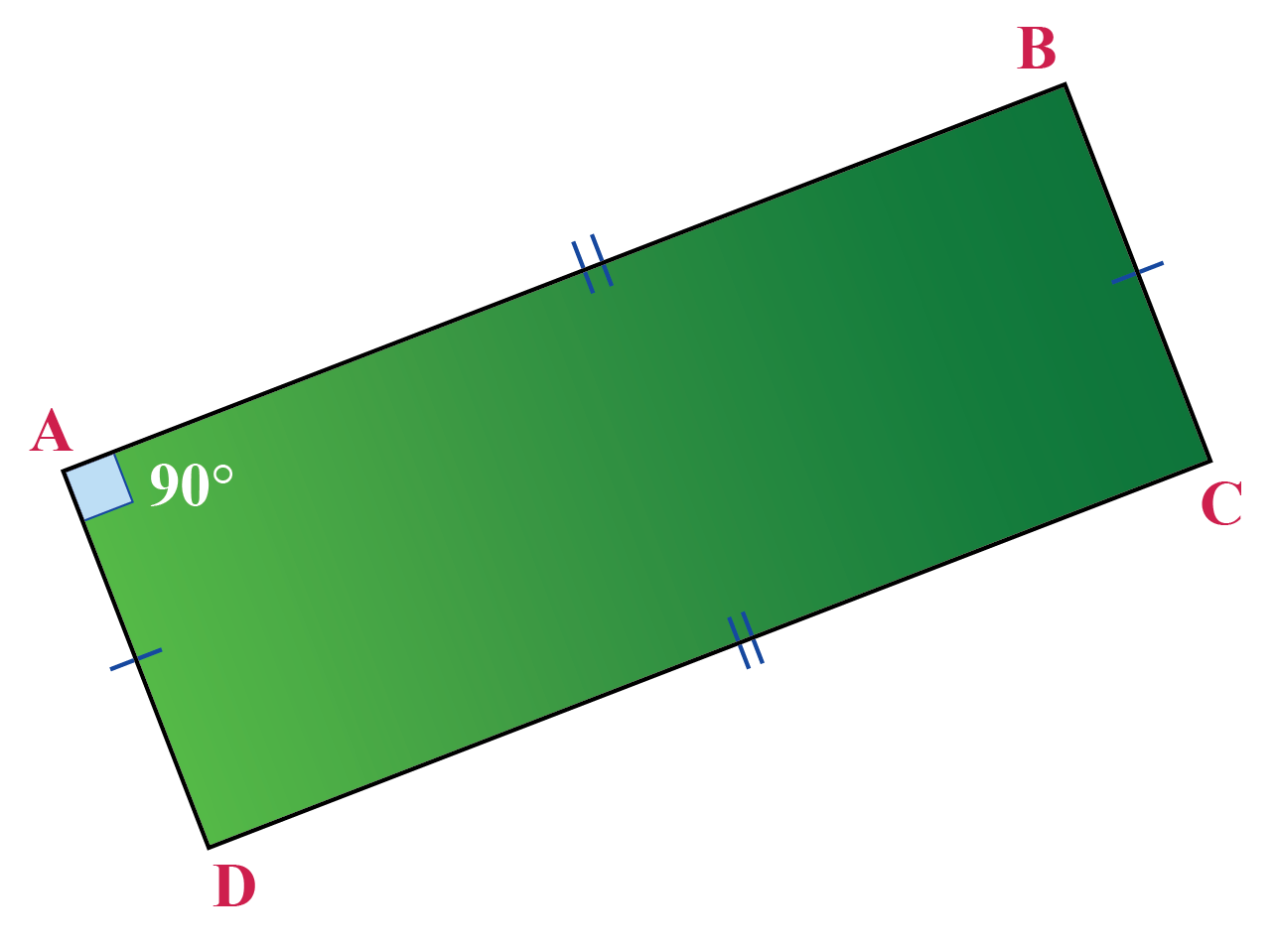Solution:

Applying the parallelogram properties we have learnt, we know that:

• the opposite angles are equal

\begin{align} \therefore \angle \text{A} = \angle \text{C} = 90^\circ \end{align}

• the adjacent angles are supplementary

\begin{align} \angle \text{A} +\angle \text{D} &= 180^\circ \\ \angle\text{D} &= 180^\circ -90^\circ (\because \angle\text{A} = 90^\circ \: \text{given} )\\ \therefore \angle\text{D} &= 90^\circ\ \\ \Rightarrow \angle \text{B} &=90^\circ \end{align}

 \begin{align}\angle\text{A}=\angle\text{B}=\angle\text{C}=\angle\text{D}= 90^\circ\end{align}
 Example 2

Prove that when the parallelogram diagonals bisect each other at $$90^\circ$$, it is a rhombus.

Solution:

Consider the parallelogram $$\text{ABCD}$$.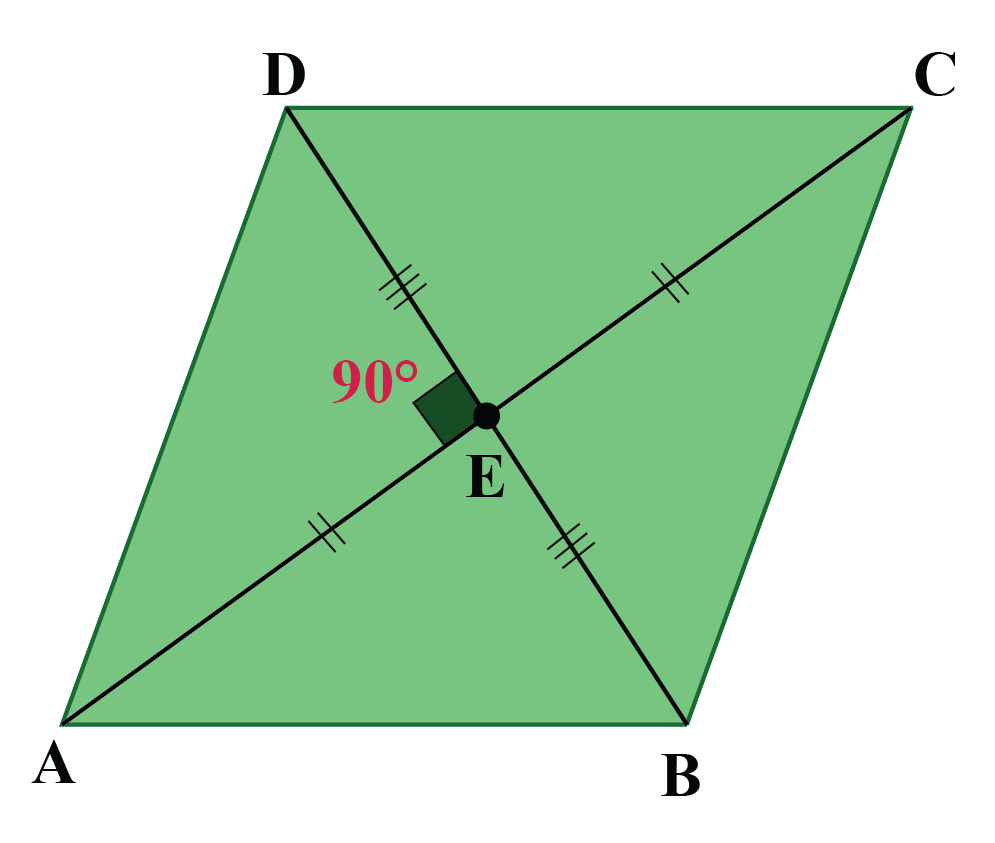\begin{align} \Delta \text{AEB}\:\text{and}\: \Delta \text{AED} \\ AE&=AE\: (\text{common})\\ BE&=ED \:(\text{given})\\ \angle AEB&=\angle AED=\,90^\circ\ (\text{given} ) \end{align}

Therefore, by SAS Congruency, $$\Delta AEB$$ and $$\Delta AED$$ are congruent.

\begin{align} \Rightarrow \text{AB = AD} \end{align}

Similarly,

Considering  $$\Delta \text{AED}\:\text{and}\: \Delta \text{CED}$$

\begin{align} \Rightarrow \text{AD = DC} \end{align}

Which further implies,

\begin{align}\boxed{ AB=BC=CD=AD} \end{align}

 $$\therefore$$The given parallelogram is a rhombus.
 Example 3

If in a parallelogram $$\text{ABCD}$$, $$\text{AC}$$ bisects  $$\angle\text{A}$$ and $$\angle\text{C}$$, show that $$\text{AC} \perp \text{BD}$$.

Solution: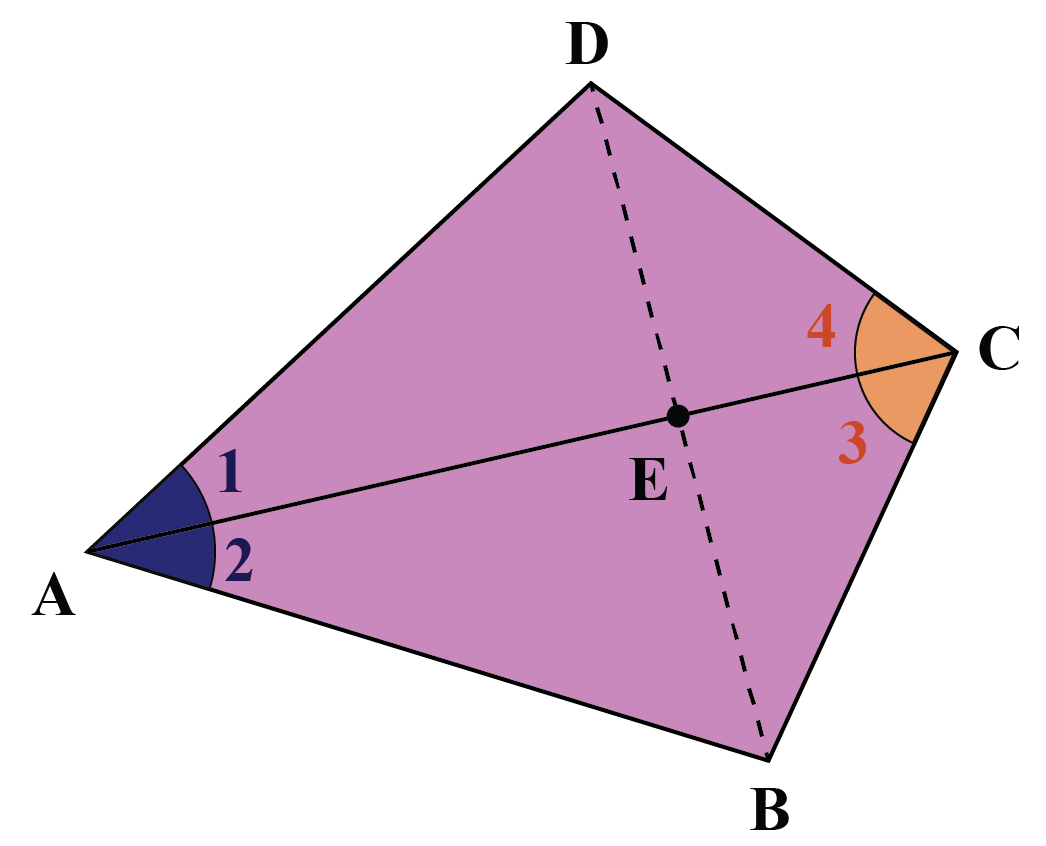\begin{align} \Delta \text{ABC}\:and\: \Delta \text{ADC}: \\ AC&=AC (\text{common}) \\ \angle 1&=\angle 2 \:( \text{given)} \\ \angle 3 &=\angle 4 \:( \text{given}) \end{align}

By the ASA criterion, the two triangles are congruent. This means that

\begin{align} \Rightarrow \text{AB = AD} \end{align}

\begin{align} \Delta \text{AEB}\:and\: \Delta \text{AED}: \\ AE&=AE (\text{common})\\ AB&=AD (\text{proved above}) \\ \angle 2&=\angle 1 \:( \text{given)} \\ \end{align}

By the SAS criterion, the two triangles are congruent.

Therefore,

\begin{align} \angle AEB = &\angle AED\\ = &\frac{1}{2} \times 180^\circ \\= &\,90^\circ \end{align}

Hence,

\begin{align}\boxed{\angle AEB=\angle AED = 90^\circ} \end{align}

 $$\therefore \;\text{AC} \perp\ \text{BD}$$
 Example 4

Find the area of a parallelogram whose base is $$7\:\text{cm}$$ and height is $$10\:\text{cm}$$.

Solution:

GivenBase $$=7\:\text{cm}$$

Height$$=10\:\text{cm}$$

Area of a parallelogram

\begin{align} &=\text{base} \times \text{height} \\ &= 7 \times 10 \\ &=70\: \text{cm}^2 \end{align}

 $$\therefore$$ Area of the parallelogram $$=70\: \text{cm}^2$$Think Tank
1. Can a kite be called a parallelogram?
2. What elements of a trapezium should be changed to make it a parallelogram?
3. Can there be a concave rhombus?

CLUEless in Math? Check out how CUEMATH Teachers will explain Parallelograms to your kid using interactive simulations & worksheets so they never have to memorise anything in Math again!

Explore Cuemath Live, Interactive & Personalised Online Classes to make your kid a Math Expert. Book a FREE trial class today!

## Practice Questions

Here are few activities for you to practice. Select/Type your answer and click the "Check Answer" button to see the result.Challenging Questions
1. Two parallel lines are intersected by a transversal. Consider a closed four-sided figure ABCD formed by the bisectors of the interior angles. Prove that ABCD is a rectangle.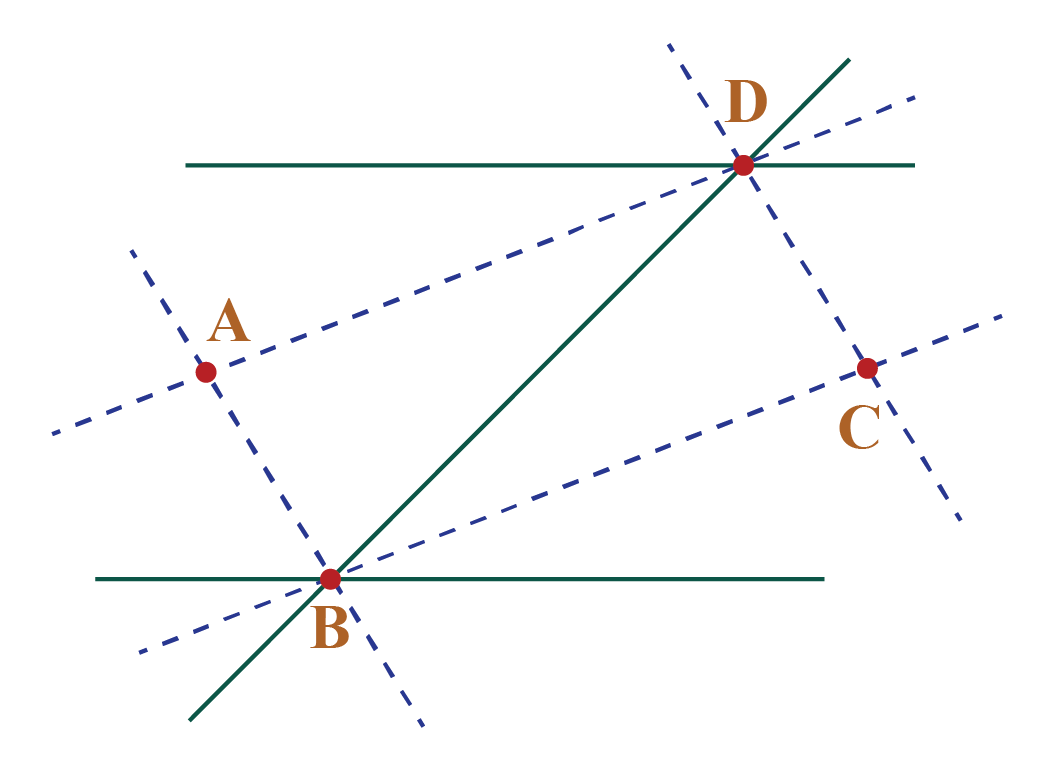IMO (International Maths Olympiad) is a competitive exam in Mathematics conducted annually for school students. It encourages children to develop their math solving skills from a competition perspective.

## 1. What are the different types of parallelograms?

Rectangle, rhombus and square are the three different types of parallelograms.

## 2. What are the various parallelogram formulas?

The various formulas for parallelograms are given here:

1. Area of the parallelogram when the base and height is known:

$$\text{base} \times \text{height}$$

2. Area of the parallelogram when the diagonals are known:

$$\frac{1}{2} \times d_{1} \times d_{2} sin (y)$$

where $$y$$ is the angle at the intersection of the diagonals

3. Area of the parallelogram using Trignometry:

$$\text{ab}$$$$sin(x)$$

where $$\text{a}$$ and $$\text{b}$$ are the length of the parallel sides and $$x$$ is the angle between the given sides of the parallelogram.

Parallelogram perimeter:

$$2 \!\times\! \text{(sum of length of adjacent sides)}$$

## 3. What is the area of a parallelogram?

The area of a parallelogram is the space occupied by the parallelogram in two-dimensional space.

grade 9 | Questions Set 1